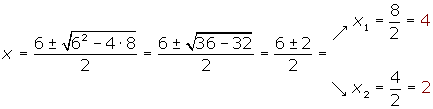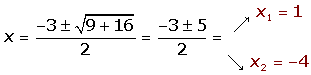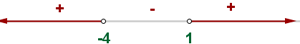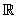Chapters

Consider the inequality:

x² − 6x + 8 > 0

It can be solved by following these steps:

1.Equal the polynomial of the first member to zero and the roots of the quadratic equation are obtained.

x² − 6x + 8 = 02. Represent these values in the real line. Take one point of each interval and evaluate the sign in each:P(0) = 0² − 6 · 0 + 8 > 0

P(3) = 3² − 6 · 3 + 8 = 17 − 18 < 0

P(5) = 5² − 6 · 5 + 8 = 33 − 30 > 0

3. The solution is defined by the intervals (or the interval) that have the same sign as the polynomial.S = (-∞, 2) (4, ∞)

x² + 2x +1 ≥ 0

x² + 2x +1 = 0(x + 1)² ≥ 0

As a number squared is always positive the solution is.

Solution
x² + 2x +1 ≥ 0(x + 1)² ≥ 0x² + 2x +1 > 0(x + 1)² > 0x² + 2x +1 ≤ 0(x + 1)² ≤ 0x = − 1
x² + 2x +1 < 0(x + 1)² < 0x² + x +1 > 0

x² + x +1 = 0When there are no real roots, give the polynomial any value if:

The sign obtained coincides with the inequality, the solution is.

The obtained sign does not coincide with that of the inequality and thus, has no solution.

 Solution x² + x +1 ≥ 0x² + x +1 > 0x² + x +1 ≤ 0x² + x +1 < 0## Example 1

7x² + 21x − 28 < 0

x² +3x − 4 < 0

x² +3x − 4 = 0P(−6) = (−6)² +3 · (−6)− 4 > 0

P(0) = 0² +3 · 0 − 4 < 0

P(3) = 3² +3 · 3 − 4 > 0(−4, 1)

## Example 2

−x² + 4x − 7 < 0

x² − 4x + 7 = 0P(0) = −0² + 4 ·0 − 7 < 0

S =## Example 3P(−3) = 4 · (−3)² − 16 > 0

P(0) = 4 · 0 ² − 16 < 0

P(3) = 4 · 3 ² − 16 > 0(-∞, −2] [2, +∞)

Did you like the article?(No Ratings Yet)Loading...

Emma

I am passionate about travelling and currently live and work in Paris. I like to spend my time reading, gardening, running, learning languages and exploring new places.

Did you like
this resource?

Bravo!